calculus. We will assume we have a particular function of x, such that If x is assigned the value 10, the corresponding value of y will be (10)2 or 100. Now, if we increase the value of x by 2, making it 12, we may call this increase of 2 an increment or x. This results in an increase in the value of y, and we may call this increase an increment or y. From this we write"> Increments and DifferentiationCustom SearchINCREMENTS AND DIFFERENTIATION In this section we will extend our discussion of limits and examine the idea of the derivative, the basis of differential calculus. We will assume we have a particular function of x, such that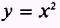If x is assigned the value 10, the corresponding value of y will be (10)2 or 100. Now, if we increase the value of x by 2, making it 12, we may call this increase of 2 an increment orx. This results in an increase in the value of y, and we may call this increase an increment ory. From this we write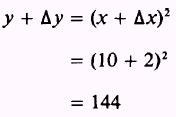As x increases from 10 to 12, y increases from 100 to 144 so thatand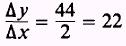We are interested in the ratiobecause the limit of this ratio asx approaches zero is the derivative of y = f(X) As you recall from the discussion of limits, asx is made smaller,y gets smaller also. For our problem, the ratioapproaches 20. This is shown in table 4-1. Table 4-l.-Slope ValuesWe may use a much simpler way to find that the limit of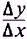asx approaches zero is, in this case, equal to 20. We have two equationsand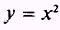By expanding the first equation so that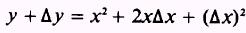and subtracting the second from this, we have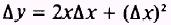Dividing both sides of the equation byx gives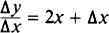Now, taking the limit asx approaches zero, gives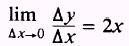Thus,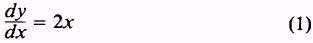NOTE: Equation (1) is one way of expressing the derivative of y with respect to x. Other ways are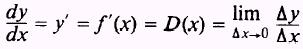Equation (1) has the advantage that it is exact and true for all values of x. Thus if x=10 then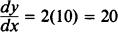and if x=3 thenThis method for obtaining the derivative of y with respect to x is general and may be formulated as follows: 1. Set up the function of x as a function of (x +x) and expand this function. 2. Subtract the original function of x from the new function of (x +x). 3. Divide both sides of the equation byx. 4.Take the limit of all the terms in the equation asx approaches zero. The resulting equation is the derivative of f(x) with respect to x.Integrated Publishing, Inc. - A (SDVOSB) Service Disabled Veteran Owned Small Business